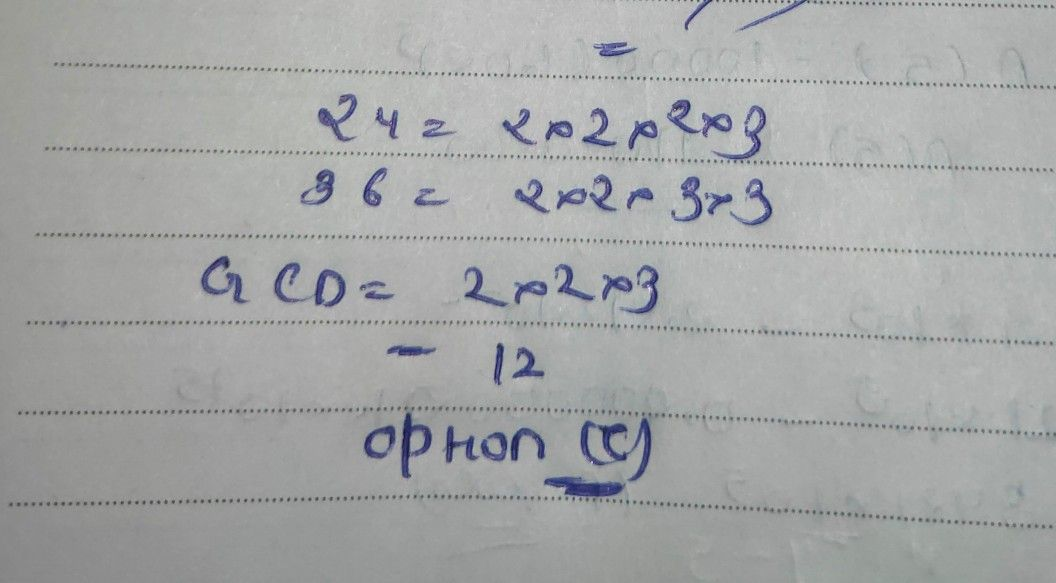Symbol
Problemof 24 and 367 1 What is the greatest common facCtor . 12 D. 24 A. 4 B 8 2. What is the greatest common factor of the variables of $12x^{5}y^{4}+16x^{3}2$ A. x*y* B y C x3 D x * What is the greatest common factor of the numerical coefficients $ot6y^{2}+9y$ A. 2 B. 3 C 4 D. 35 7 4. What is the factored form of the expression in no. A. 2y (2y + 3) B. 3y (2y+3) C 4y (2y + 3) D. 5y (2y+ 3) 5. What is the factored form of the expression 4x + 167 A. 4(x + 2) B 4(x + 3) C. 4(x+4) D. 4(x+5) 6 Which of the following is the factored form of 50x2y5- 75x y ? A 25(2x 3x'y") C 25x y (2- 3x'y) B. B 25x y (2x- 3x'y) D 25x3y (2-4x'y) 7. What is the missing term in 8x+ C 10x = 8x ( x +2 )? A. 4x B. 6x D. 16x 8. Which of the following is the product of 4m² (3m + 4)? 16m3 C 12m3 + 16m2 D. $16m^{3}+12m$ A. $12m+16m$ B. 12m3 + 9 Which of the following has factors (2x - y)and (2x + y)? A. 2x2 + y? B 2x?- y? C. 4x? + y2 D. 4x2 - y? 10. Using the pattern for factoring the sum of cubes, what is the factored form of 8 + b32 A $\left(2-b\right)\left(4-2b+b^{2}\right)$ $\left(2-b\right)\left(4+2b+b^{2}\right)$ $C$ $\left(2+b\right)\left(4-2b+b^{2}\right)$ $D\left(2+b\right)\left(4+2b+b^{2}\right)$ B 11.If one factor of a perfect square trinomial is 2xy - 3x, what is the other factor? A. 2y - 3x B 2y + 3x $C2xy-3x$ $D2xy+3x$ 12 Which of the following is a perfect square number? A 8 B. 24 C 125 D 121 13 All of the following expressions are perfect squares, EXCEPT one What is it? b2 B x'y6 C. 16a?b6 D. 25x y@ A. 12a 14 Which of the following is equal to x? - $6xy+9y^{2}3$ E A (x-3y)? $B\left(x+3y\right)$ $\left(2x-3y\right)^{2}$ $D$ $\left(2x$ Which of the following is the complete factored form of $+3y\right)^{2}$ $8x^{2}-24xy+18y^{2}7$ $A$ $2\left(2x-3y\right)\left(2x-$ 3y) $C$ $2\left(3x+2y\right)^{2}$ $D$ $2\left(3x-2y\right)$ $B$ $2\left(2x-3y\right)\left(2x+3y\right)$ 16 $Mnicnottnefo|lomnG$ trinomials has factors A B $6a^{2}-7b+2b^{2}$ $6a^{2}+7b-2b^{2}$ D. $rs\left(3a-2b\right)and\left(2a-b\right)7$ $6a^{2}-7b-2b^{2}$ $6a^{2}+7b+2b^{2}$
7th-9th grade
Algebra
Search count: 119
SolutionQanda teacher - Askhina